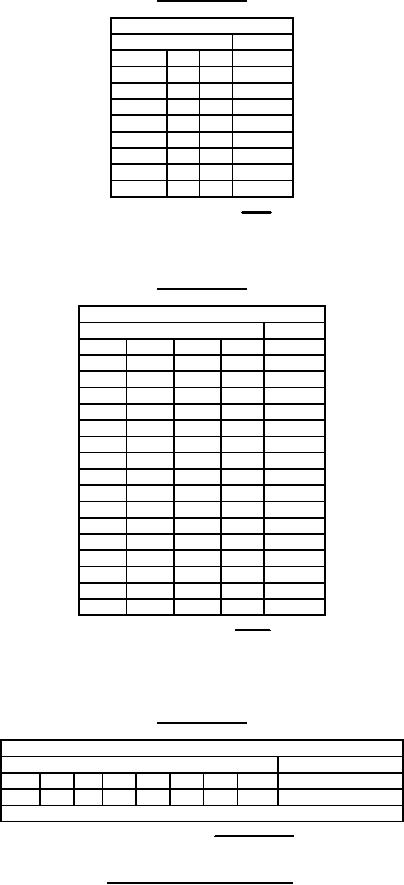Click here to make tpub.com your Home Page Page Title: Figure 2. Truth tables and logic equations (Device type 04) Back | Up | NextWeb www.tpub.comHome

Information Categories
Aerographer
Automotive
Aviation
Construction
Diving
Draftsman
Engineering
Electronics
Food and Cooking
Logistics
Math
Medical
Music
Nuclear Fundamentals
Photography
ReligionMIL-M-38510/650B
Device type 02
Truth table each gate
Input
Output
A
B
C
Y
L
L
L
H
H
L
L
H
L
H
L
H
H
H
L
H
L
L
H
H
H
L
H
H
L
H
H
H
H
H
H
L
Positive logic Y = ABC
Device type 03
Truth table each gate
Input
Output
A
B
C
D
Y
L
L
L
L
H
H
L
L
L
H
L
H
L
L
H
H
H
L
L
H
L
L
H
L
H
H
L
H
L
H
L
H
H
L
H
H
H
H
L
H
L
L
L
H
H
H
L
L
H
H
L
H
L
H
H
H
H
L
H
H
L
L
H
H
H
H
L
H
H
H
L
H
H
H
H
H
H
H
H
L
Positive logic Y = ABCD
Device type 04
Truth table
Inputs
Output
A
B
C
D
E
F
G
H
Y
H
H
H
H
H
H
H
H
L
All other combinations of H and L at the inputs give a H output
Positive logic Y = ABCDEFGH
FIGURE 2. Truth table and logic equations - Continued.
15